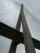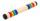# Car

Car travels 1/3 of the trip on the first day, second day 2/5 of the trip and left even 340 km for next days. How long is the trip?

Result

x =  1275 km

#### Solution:

$x-\dfrac{ 1}{ 3} x - \dfrac{ 2}{ 5} x = 340 \ \\ x(1 - \dfrac{ 1}{ 3} - \dfrac{ 2}{ 5}) = 340 \ \\ x = \dfrac{ 340} {1 - \dfrac{ 1}{ 3} - \dfrac{ 2}{ 5}} \ \\ x = 1275 \ \text { km }$

Leave us a comment of this math problem and its solution (i.e. if it is still somewhat unclear...):Be the first to comment!#### Following knowledge from mathematics are needed to solve this word math problem:

Need help calculate sum, simplify or multiply fractions? Try our fraction calculator. Do you have a linear equation or system of equations and looking for its solution? Or do you have quadratic equation? Do you want to convert length units?

## Next similar math problems:

1. Grandmother and grandfatherGrandmother baked cakes. Grandfather ate half, then quarter of the rest ate Peter and Paul ate half of rest. For parents left 6 cakes. How many cakes maked the grandmother?
2. PearsThere were pears in the basket, I took two-fifths of them, and left six in the basket. How many pears did I take?
3. WithdrawalIf I withdrew 2/5 of my total savings and spent 7/10 of that amount. What fraction do I have in left in my savings?
4. Equation 29Solve next equation: 2 ( 2x + 3 ) = 8 ( 1 - x) -5 ( x -2 )
5. Bridge piersOne quarter of the bridge pier is sunk into the ground. Two thirds are in the water. Protruding above the water is 1.20 m long. Determine the height of bridge piers.
6. BarHalf of the bar is green, the third is yellow and the remaining 40cm is red. How long is a bar?
7. TreesFrom the total number of trees in the orchard, there are two-fifths pearls and apples are three eighty. The rest of the trees are 9 ceremonial. How many trees are in the set?
8. FruitsAmy bought a basket of fruits 1/5 of them were apples,1/4 were oranges, and the rest were 33 bananas. How many fruits did she buy in all?
9. TimeageSeven times of my age is 8 less than the largest two-digit number. How old I am?
10. Equation 15Solve equation with variables on both sides:
11. NumberI think the number. If I add to its third seven I get same as when to its quarter add 8. Which is the number?
12. Negative in equation2x + 3 + 7x = – 24, what is the value of x?
13. EquationFind x: x + 1/2 = 1/3
14. Family 8Father is 38 years old, daughter 12, son 14. How many years will father have as many years as his children together?
15. Simple equation 8Solve the following equation: 36=-(1+7x)-6(-7-x)
16. Missing numberBlank +1/6 =3/2 find the missing number
17. Simple equation 9Solve the following equation: -8y+5=-9y+9